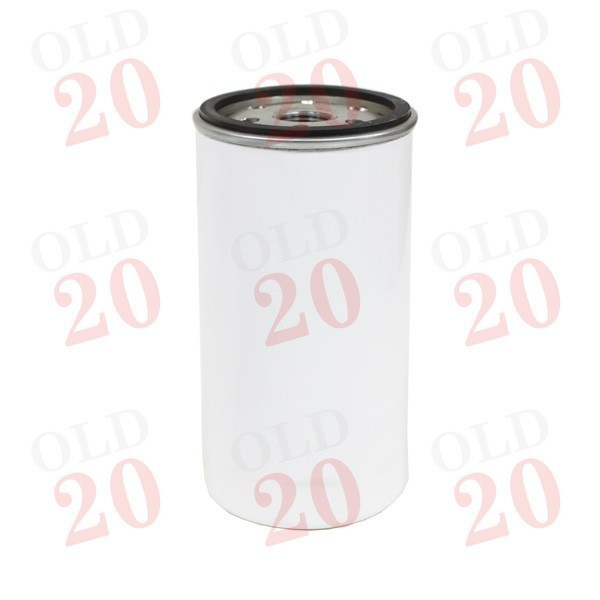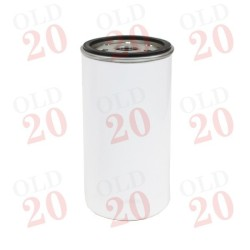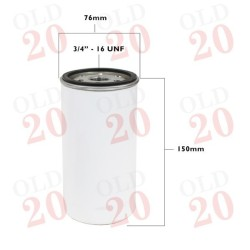••# Filter - Oil

2045003

£6.60
Tax included
QuantitySecure Online Ordering

All our pages are encrypted for your securityWorldwide Delivery

We ship nearly all the parts we supply worldwide!12 Month Warranty

All parts covered by a 12 month warranty

Length: 150mm
Outer Diameter: 78mm
Type: Spin on
2045003

Section
Filtration

### Specific References

• Tractors < Landini < 30 < 5530

• Tractors < Landini < 30 < 5830

• Tractors < Landini < 30 < 6030

• Tractors < Landini < 40 < 5840

• Tractors < Landini < 40 < 6040

• Tractors < Landini < 500 < 5500

• Tractors < Landini < 60 < 5560

• Tractors < Landini < 60 < 5860

• Tractors < Landini < 60 < 6060

• Tractors < Landini < 70 < 5870

• Tractors < Landini < 70 < 6070

• Tractors < Landini < Blizzard < Blizzard 50

• Tractors < Landini < Blizzard < Blizzard 60

• Tractors < Landini < Globus < Globus 50

• Tractors < Landini < Prima < 4000R

• Tractors < Landini < Prima < 4000Special

• Tractors < Landini < Prima < 5000DT

• Tractors < Landini < Prima < 5000R

• Tractors < Landini < Prima < 5000R-N

• Tractors < Landini < Prima < 5000R-S

• Tractors < Landini < Prima < 5000Special

• Tractors < Landini < Prima < 6000

• Tractors < Landini < Prima < 6000Special

• Tractors < Landini < Prima < 7000R

• Tractors < Landini < Prima < 7000Special

• Tractors < Landini < Prima < 8000DT

• Tractors < Landini < Prima < 8000R

• Tractors < Leyland Marshall < Leyland < 245

• Tractors < Leyland Marshall < Leyland < 253

• Tractors < Leyland Marshall < Marshall < 502

• Tractors < Leyland Marshall < Marshall < 602

• Tractors < Leyland Marshall < Marshall < 604

• Tractors < Leyland Marshall < Marshall < 702

• Tractors < Leyland Marshall < Marshall < 704

• Tractors < Leyland Marshall < Marshall < 802

• Tractors < Leyland Marshall < Marshall < 804

• Tractors < Massey Ferguson < Pre-100 Series < 35

• Tractors < Massey Ferguson < Pre-100 Series < 65

• Tractors < Massey Ferguson < 100 Series < 133

• Tractors < Massey Ferguson < 100 Series < Various MF100 Series < 134V

• Tractors < Massey Ferguson < 100 Series < 148

• Tractors < Massey Ferguson < 100 Series < 152

• Tractors < Massey Ferguson < 100 Series < Various MF100 Series < 152S

• Tractors < Massey Ferguson < 100 Series < 154

• Tractors < Massey Ferguson < 100 Series < Various MF100 Series < 154S

• Tractors < Massey Ferguson < 100 Series < Various MF100 Series < 155

• Tractors < Massey Ferguson < 100 Series < 158

• Tractors < Massey Ferguson < 100 Series < Various MF100 Series < 164TS

• Tractors < Massey Ferguson < 100 Series < 165

• Tractors < Massey Ferguson < 200 Series < 230

• Tractors < Massey Ferguson < 200 Series < Various < 233

• Tractors < Massey Ferguson < 200 Series < Various < 234S

• Tractors < Massey Ferguson < 200 Series < Various < 235

• Tractors < Massey Ferguson < 200 Series < 240

• Tractors < Massey Ferguson < 200 Series < Various < 240S

• Tractors < Massey Ferguson < 200 Series < Various < 245

• Tractors < Massey Ferguson < 200 Series < 250

• Tractors < Massey Ferguson < 200 Series < Various < 253

• Tractors < Massey Ferguson < 200 Series < Various < 254

• Tractors < Massey Ferguson < 200 Series < Various < 254S

• Tractors < Massey Ferguson < 200 Series < Various < 255

• Tractors < Massey Ferguson < 200 Series < Various < 260

• Tractors < Massey Ferguson < 200 Series < Various < 264TS

• Tractors < Massey Ferguson < 200 Series < Various < 265S

• Tractors < Massey Ferguson < 200 Series < Various < 285S

• Tractors < Massey Ferguson < 300 < Various < 333

• Tractors < Massey Ferguson < 300 < 340

• Tractors < Massey Ferguson < 300 < Various < 342

• Tractors < Massey Ferguson < 300 < 350

• Tractors < Massey Ferguson < 300 < Various < 352

• Tractors < Massey Ferguson < 300 < Various < 353

• Tractors < Massey Ferguson < 300 < Various < 353LX

• Tractors < Massey Ferguson < 300 < Various < 353S

• Tractors < Massey Ferguson < 300 < Various < 354GE

• Tractors < Massey Ferguson < 300 < Various < 354S

• Tractors < Massey Ferguson < 300 < Various < 355

• Tractors < Massey Ferguson < 300 < 360

• Tractors < Massey Ferguson < 300 < Various < 363

• Tractors < Massey Ferguson < 300 < Various < 363LX

• Tractors < Massey Ferguson < 300 < Various < 364GE

• Tractors < Massey Ferguson < 300 < Various < 364S

• Tractors < Massey Ferguson < 300 < Various < 374H

• Tractors < Massey Ferguson < 300 < Various < 394H

• Tractors < Massey Ferguson < 500 < 550

• Tractors < Massey Ferguson < 500 < 560

• Tractors < Ursus < Various < 2802

• Tractors < Ursus < Various < 2812

• Tractors < Ursus < Various < 3502

• Tractors < Ursus < Various < 3512

• Tractors < Ursus < Various < 3514

• Tractors < Ursus < Various < MF231

• Tractor Sections < Engine < Lubrication

• Tractor Sections < Filtration < Engine Oil Filter

AHM2094, AKU1033, 2654408, 1447082M1, 2654156, 30270, 1447082M91